# 第五章：图（图的遍历操作）

## 1.图的遍历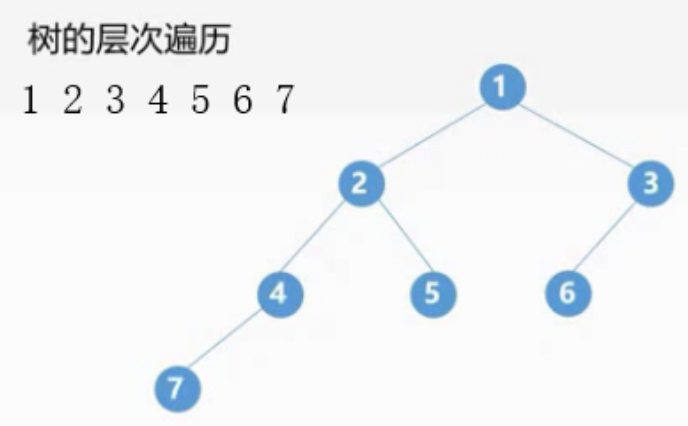## 2.广度优先搜索(BFS)

• 首先访问起始顶点v
• 接着由v出发依次访问v的各个 未被访问过 的邻接顶点w1,w1....wi
• 然后依次访问w1,w2....wi的所有 未被访问过 的邻接顶点
• 在从这些访问过的顶点出发,访问它们所有 未被访问过 的邻接顶点
• 以此类推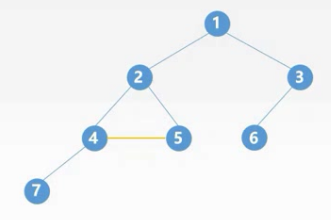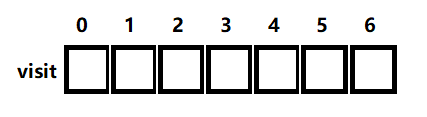bool visited[MAX_TREE_SIZE]
void BFSTraverse(Graph G){
for(int i=0; i<G.vexnum;i++){
visited[i]=FALSE;
}
InitQueue(Q);
//for循环的作用，我们上面讲的是一个连通图，所有顶点都可以通过一个顶点依次进行访问
//但是如果一个图不是连通的，我们需要遍历所有顶点
for(int i=0;i<G.vexnum;i++){
if(!visited(i)){
BFS(G,i);
}
}
}
//广度优先搜索
void BFS(Graph G,int V){
visit(v); //访问
visited=TRUE; //TRUE 入队了，FALSE未入队
EnQueue(Q,v); //将结点入队
while(!isEmpty(Q)){ //判断队列是否是空
DeQueue(Q,v); //出队队首元素，并赋值到v中
//FirstNeighbor 求图G中顶点x的第一个邻接顶点，存在返回顶点号，不存在返-1
//判断 w是否大于0，如果是-1则说明没有邻接顶点了
//NextNeighbor 求图G中顶点v的的下一个邻接顶点并赋值给w
for(int w=FirstNeighbor(G,v);w>0;w=NextNeighbor(G,v,w)){
if(!visited[w]){//判断是否入队过
visit[w];
visited[w]=TRUE;
EnQueue(Q,w);
}
}
}
}


## 3.应用

### 3.1无权图单源最短路径问题

//和广度优先搜索相似，增加了保存最短路径的一个数组
void BFS_MIN_Distance(Graph G,int u){//传入图 和 初始顶点
for(int i=0;i<G.vexnum;++i){ //
d[i]=MAX; //保存最短路径的值，我们初始化为最大值
}
visited[u]=TRUE;//标识为该顶点已经入队
d[u]=0;//初始顶点路径值改为0
EnQueue(Q,u);//入队
while(!isEmpty(Q)){//判断队列时候为空
DeQueue(Q,u);//出队队首元素
//FirstNeighbor 求图G中顶点x的第一个邻接顶点，存在返回顶点号，不存在返-1
//判断 w是否大于0，如果是-1则说明没有邻接顶点了
//NextNeighbor 求图G中顶点v的的下一个邻接顶点并赋值给w
for(int w=FirstNeighbor(G,u);w>0;w=NextNeighbor(G,u,w)){
if(!visit[w]){//判断该顶点是否已经被访问过
visited[w]=TRUE;//设置被访问过
//d[u]表示到初始顶点的最短路径，w是它的邻接点，所以+1等目前w到初始顶点的最短路径
d[w]=d[u]+1;
EnQueue(Q,w);//入队
}
}
}
}


### 3.2广度优先生成树

连通图：任意两个结点都是连通的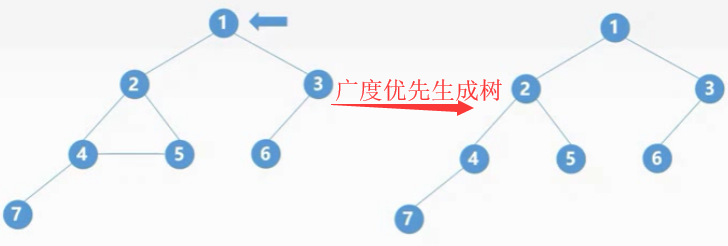## 4.深度优先搜索(DFS)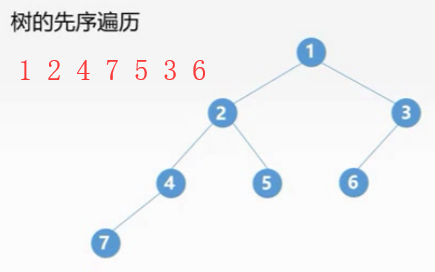• 首先访问起始顶点v
• 接着由v出发访问v的任意一个邻接且未被访问的邻接顶点Wi
• 然后再访问与Wi邻接且未被访问的任意顶点Yi
• 若Wi没有邻接且未被访问的顶点时,退回到它的上一层顶点v
• 重复上述过程,直到所有顶点被访问为止bool visited[MAX_TREE_SIZE]//辅助标记数组
void DFSTraverse(Graph G){
for(int i=0;i<G.vexnum;i++){
visited[i]=FALSE;//初始化所有结点都未必访问
}
for(int i=0;i<G.vexnum;i++){
if(!visited[i]){//如果结点未被访问
DFS(G,i);//G：图，i：起始顶点的编号
}
}
}
void DFS(Graph G,int v){
visit(v); //访问
visited[v]=TRUE;//置为访问过
for(int w=FirstNeighbor(G,v);w>0;w=NextNeighbor(G,v,w)){
if(!visit[w]){//判断该顶点是否已经被访问过
DFS(G,w);//递归
}
}
}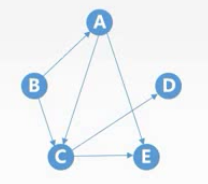## 5.深度优先生成树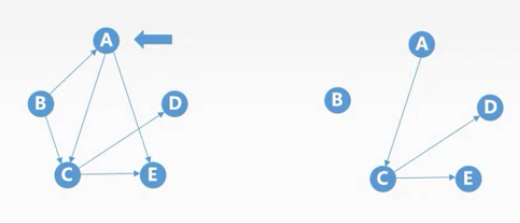## 6.遍历与连通性问题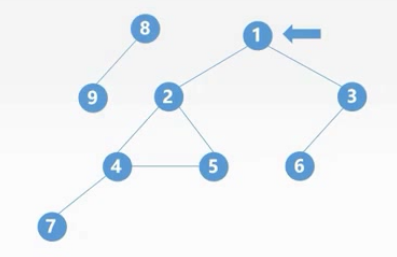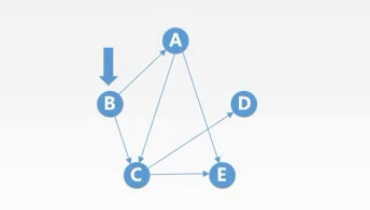## 7.理木客